• 建立回归模型的一般步骤如下图 1、具体（社会经济）问题 当我们想去解决一些现实生活、经济问题时，需要将具体问题量化成数据，然后通过观察与揭示事物（数据）之间的内在联系得出规律，从而达到解决现实...
文章福利：Python学习精选书籍10本

建立回归模型的一般步骤如下图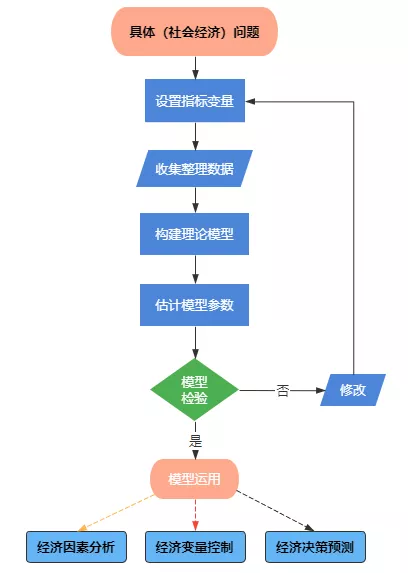1、具体（社会经济）问题
当我们想去解决一些现实生活、经济问题时，需要将具体问题量化成数据，然后通过观察与揭示事物（数据）之间的内在联系得出规律，从而达到解决现实经济问题（及时止损、预测），奔着这个目标产生了一些列的可行性问题。

2、设置指标变量（量化具体问题）
可行性问题已经产生，接下来就要根据问题研究的目的设置因变量 y ，然后选取一些和因变量y有统计关系的自变量 x1、x2...
这里一定要明确一点：什么是因变量 y 和自变量 x，其实通俗来理解就是下图中的因果关系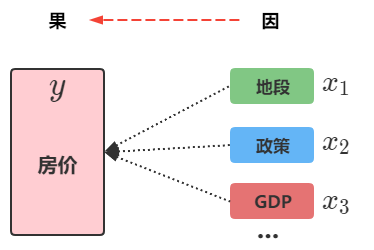我们需要通过经济变量（要研究的） '果' 选出一些影响它且合理的变量 '因',在回归模型中对于这两类变量有一些术语需要了解
因变量（果）也被称为被解释变量、内生变量；
自变量（因）也被称为解释变量、外生变量；
注意01：在变量选择这一块，研究不同的问题领域跨度大、专业性强，对于完全不了解的领域需要和这方面的专家、或者有经验的人士询问合作。这样可以更好的帮助我们确定研究问题的模型变量，做到不耻下问 。
注意02：回归模型的解释变量选取个数上并不是越多越好，若选取的变量之间有较大的信息重叠，就会出现共线性问题，并且变量多导致的计算量大，误差也大，进而导致最终模型参数精度也不高。
一般在选取指标变量时往往不能一次完全正确，需要反复调整找到最合适的指标变量。

3、收集、整理数据
指标确定之后，接下来就是搜集需要的指标数据了。要搜集样本数据可分为时间序列数据和横截面数据。
时间序列数据：
时间序列数据就是按照时间顺序排列数据，如下图，各指标数据跟着时间在变化：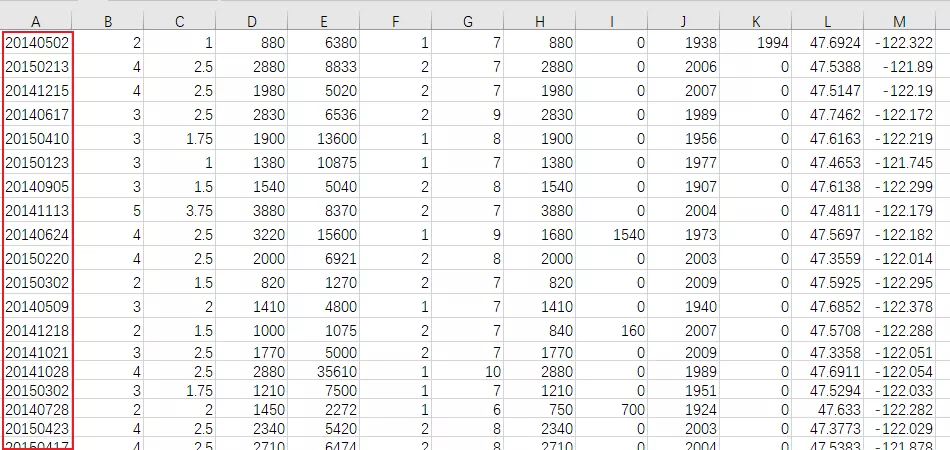横截面数据：
横截面数据即在同一时间截面上统计的数据集，如2018年我国各省市GDP数据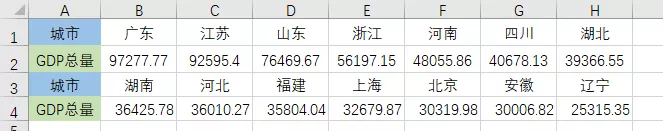注意点：时间序列数据容易产生随机误差项的序列相关（处理方法：差分法）

4、回归模型的确定
上一步骤中，数据已经准备好，接下来就要从这些数据中提炼出一套宇宙公式--回归模型（数学形式）。首先第一步我们需要在笛卡尔坐标系中画出这些样本点的散点图，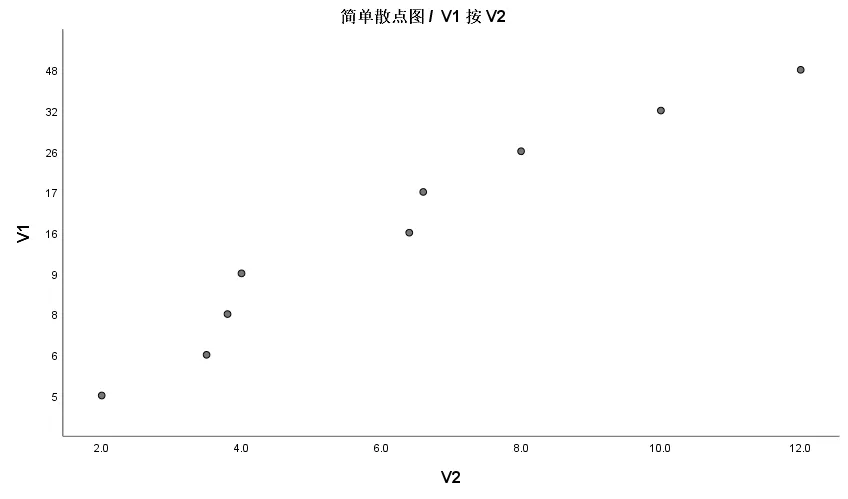为什么要先画散点图呢？我们知道回归模型的种类有多种，如线性回归、非线性回归...。画出散点图，我们可以根据散点图的分布形式大致确定该建立哪一种回归模型才是较合适的。
如果根据散点图实在无法确定模型的形式时，则可以将有争议的模型分别进模拟，然后从模拟结果中选出模拟效果最好的一个作为最终的理论回归模型。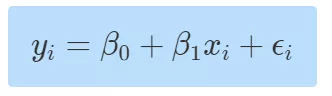5、模型参数估计
当回归模型的的具体分类选取确定后，接下来就要对模型中的未知参数进行估计，常见的也是最最经典的参数估计方法为：最小二乘法。
在最小二乘法的基础上又衍生出了偏最小二乘法、主成分回归、岭回归等，他们都是为了解决不满足模型基本假设而衍生出来的新方法。

6、模型检验与修改
初步的回归模型建立好后，还不能直接用于实际应用，模型是否正确解释问题指标之间的因果关系还是个未知数，此时需要去检验模型的可行性。
通常对模拟有效性的检验有两种方法：
1 统计性检验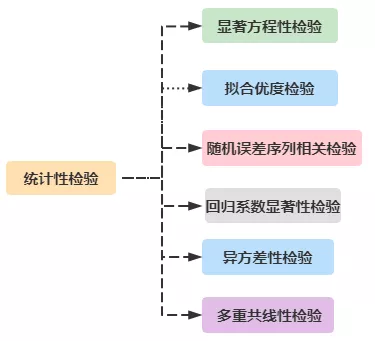2 具体问题（经济）意义检验
模型侧面揭示了具体的社会经济问题，例如我国GDP增长量与银行贷款发放量、耗电量等之间的关系从经济理论是上看是正相关关系，但是模型中的回归系数若为负数，则这个模型也是没有意义的。造成这种现象的原因可能有：自变量之间存在多重共线性、数据质量问题等。
模型需要通过统计检验和经济意义检验共同检验通过后才是有效可行的。

7、回归模型的应用
经过以上的种种九九八十一难，终于可以将该模型用于实际生活、经济问题上了。
我们可以从模型的回归系数上发现所研究的变量之间的结构关系，从而给出量化后的评价与建议。
调控：确定好的回归模型反馈了经济变量之间的因果关系后，根据已知结果的情况下调整具体的经济指标数据等。
例如：为了降低通货膨胀指标为5%以下，可以根据回归模型确定货币发行量、银行的存款利率等。
预测：可以根据回归模型预测我国2022年的国民收入等。
以上几个步骤就是一个回归模型建立到使用的全部流程，模型的修改往往要反复修正后才能得到一个理想模型。这个反反复复修改模型的过程可以从写论文中感触到。
--- END ---
更多精彩内容请关注 公众号：数据与编程之美
原文地址：建立回归模型的完整步骤
展开全文统计学
• 建立统计回归模型的基本步骤Linear Regression and Regression Trees 线性回归和回归树 by Satoru Hayasaka and Rosaria Silipo, KNIME 由 悟早坂 和 罗萨丽娅Silipo， 尼米 When we talk about Machine Learning ...

建立统计回归模型的基本步骤

Linear Regression and Regression Trees
线性回归和回归树
by Satoru Hayasaka and Rosaria Silipo, KNIME
由 悟早坂 和 罗萨丽娅Silipo，   尼米
When we talk about Machine Learning algorithms, we often think of classification problems. Indeed, the most common problems in machine learning are about classification, mainly because predicting a few classes is often easier than predicting an exact number. A less commonly used branch of data science involves numerical predictions. A family of algorithms dedicated to solving numerical prediction problems is regressions, in their basic and ensemble form. In this article, we describe two basic regression algorithms: linear regression and regression tree.
当我们谈论机器学习算法时，我们经常想到分类问题。 确实，机器学习中最常见的问题是关于分类的，这主要是因为预测几个类别通常比预测准确的数字容易。 数据科学中较少使用的分支涉及数值预测 。 专用于解决数值预测问题的一系列算法是基本形式和整体形式的回归 。 在本文中，我们描述了两种基本的回归算法： 线性回归和回归树 。
数值预测问题 (The problem of numeric predictions)
An overarching goal of regression analysis is to model known numerical outcomes based on the available input features in the training set. Classic case studies are stock price prediction, demand prediction, revenue forecasting, and even anomaly detection . Most forecasting and prediction problems generally require numerical outcomes.
回归分析的总体目标是根据训练集中可用的输入特征对已知的数值结果进行建模。 经典案例研究包括股票价格预测 ， 需求预测 ， 收入预测 ，甚至异常检测 。 大多数预测和预测问题通常需要数值结果。
Many algorithms have been proposed over the years, and, among those — many regression algorithms. Two very basic classic and widely adopted regression algorithms are linear regression and regression tree. We want to explore the theory behind each one of them and their pros and cons, to better understand when it is better to use one rather than the other.
这些年来，已经提出了许多算法，其中包括许多回归算法。 线性回归和回归树是两个非常基本的经典且被广泛采用的回归算法。 我们希望探索其中每一个背后的理论及其优缺点，以更好地理解何时使用一种而非另一种更好。
Let’s take a toy example to run our exploration: a small dataset, two numeric features (one is the target, one is the input). The “auto-MPG” dataset from the UC Irvine Repository provides a description of 398 car types, by brand, engine measures, and chassis features. Two of these attributes sound interesting for our little experiment: Horsepower (HP) and mileage per gallon (MPG) (Figure 1). It is likely that the two attributes are related.
让我们以一个玩具示例进行探索：一个小的数据集，两个数字特征(一个是目标，一个是输入)。 UC Irvine储存库中的“ auto-MPG”数据集按品牌，发动机尺寸和底盘特征提供了398种汽车类型的描述。 在我们的小实验中，其中两个属性听起来很有趣：马力(HP)和每加仑行驶里程(MPG)(图1)。 这两个属性很可能是相关的。
Is it possible to build a regression model where MPG (outcome y) can be described through HP (input feature x)? The goal of the regression model is to build that function f(), so that y=f(x).
是否可以建立一个可以通过HP(输入特征x )描述MPG(结果y )的回归模型？ 回归模型的目标是构建该函数f() ，以便y = f(x) 。
线性回归 (Linear Regression)
There are different approaches to regression analysis. One of the most popular approaches is linear regression , in which we model the target variable y as a linear combination of input features x.
回归分析有不同的方法。 线性回归是最流行的方法之一，其中我们将目标变量y建模为输入特征x的线性组合。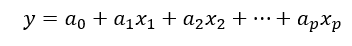If there is only one input feature, the resulting model describes a regression line. If there are more than one input features, the model describes a regression hyperplane.
如果只有一个输入要素，则生成的模型将描述一条回归线。 如果有多个输入要素，则模型将描述回归超平面。
Figure 2 is an example of a linear regression model in a two-dimensional space. The slope and the intercept of the regression line are controlled by the regression coefficients.
图2是二维空间中线性回归模型的示例。 回归线的斜率和截距由回归系数控制。
Figure 2. A linear regression model fitting MPG (y) from HP (x) on the Auto-MPG dataset.

Fitting a linear regression model means adjusting the regression coefficients to best describe the relationship between x and y. To do so, we calculate the total error between the observed data and the linear regression predictions. The single error at each data point is referred to as a residual. The best model minimizes the total error, i.e. the residuals for all data points simultaneously.
拟合线性回归模型意味着调整回归系数以最好地描述x和y之间的关系。 为此，我们计算观察到的数据与线性回归预测之间的总误差。 每个数据点的单个错误称为残差。 最佳模型可以最大程度地减少总误差，即所有数据点的残差同时出现。
The sum of squared residuals E is adopted as the total error:
残差平方和E被用作总误差：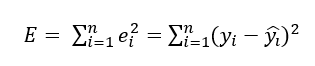as the sum across all n data points in the training set of the difference between the real value of target y and the estimated value by the linear regression model. Such difference is the residual of the data point.
表示训练集中所有n个数据点的总和，即目标y的实际值与线性回归模型的估计值之差。 这种差异是数据点的残差。
So, the regression coefficients for the linear regression model are found by minimizing the sum of squared residuals E. We are in luck and this optimization problem has a closed form solution . The problem of the optimum regression coefficients is solved in a two-dimensional space by the following formula:
因此，通过最小化残差平方和E来找到线性回归模型的回归系数。 我们很幸运，这个优化问题有一个封闭式解决方案。 通过以下公式可在二维空间中解决最佳回归系数的问题：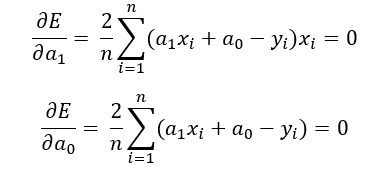这导致：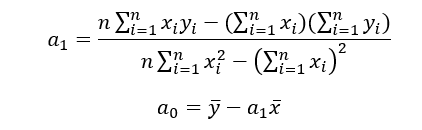Moving to a high-dimensional space, the solution to the equation system takes the form:
移至高维空间，方程组的解采用以下形式：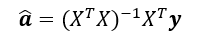Where y is the vector of target outcomes for all data rows in the training set, X the matrix of all data rows in the training set. The result is the vector of estimated regression coefficients.
其中y是训练集中所有数据行的目标结果的向量，X是训练集中所有数据行的矩阵。 结果是估计回归系数的向量。
回归树 (Regression Tree)
Another popular regression approach came out in the 90s and it is known as CART (Classification And Regression Trees) .
另一种流行的回归方法是在90年代问世的，它被称为CART(分类和回归树)。
Instead of fitting all data simultaneously as in the construction of a linear regression model, the regression tree algorithm fits the data piecewise, one piece after the other. In a two-dimensional space, in interval A, every x produces y=c(A); in interval B, every x produces y=c(B); and so on. A piecewise model, like this, is a regression tree. In a higher dimensional space, each interval becomes a region of the space.
与在线性回归模型的构建中同时拟合所有数据不同，回归树算法逐段拟合数据，一个接一个地拟合数据。 在二维空间中，在间隔A中，每个x产生y = c(A) ； 在间隔B中，每个x产生y = c(B) ； 等等。 像这样的分段模型是回归树。 在高维空间中，每个间隔都成为该空间的一个区域。
Figure 3. A regression tree model fitting MPG (y) from HP (x) on the Auto-MPG dataset.

In a regression tree model, as you can see in Figure 3, a constant value is fitted within each segment of the input attributes. This way, the outcome variable is modeled from the input features without explicitly using a mathematical function.
在回归树模型中，如图3所示，在输入属性的每个段中都拟合了一个常数值。 这样，无需明确使用数学函数即可根据输入要素对结果变量进行建模。
Now let’s have a look at how a regression tree model can be constructed.
现在让我们看一下如何构建回归树模型。
In the first step, we want to split the training set in two subsets. Therefore, we want to find the threshold S that best splits the input feature x in two segments. Within each segment m, the outcome y is modeled by the local mean value of y, as:
第一步，我们要将训练集分为两个子集。 因此，我们希望找到最能将输入特征x分为两个部分的阈值S。 在每个段米 ，其结果y由的Y，作为本地平均值建模：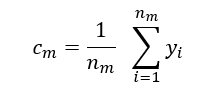Where c(m) is the constant outcome value in segment m modeled as the average value of y in segment m.
其中，c(m)为建模为y的段m的平均值值段米恒定结果值。
The constant value in each segment does not necessarily have to be the mean value, it could be anything else, like for example the quadratic average or even a function .
每个段中的恒定值不一定必须是平均值，它可以是其他任何值，例如二次平均甚至是一个函数。
In this scenario, what would be the best boundary S that splits the input feature x in two segments? Let’s first have a look at the error from such a split. Within each segment m, the error can be calculated as the sum of Euclidean distances of all points in the segment to the mean value of y, that is c(m).
在这种情况下，将输入特征x分为两部分的最佳边界S是什么？ 首先让我们看一下这种拆分产生的错误。 在每个线段m内 ，可以将误差计算为线段中所有点与y平均值(即c(m))的欧式距离之和。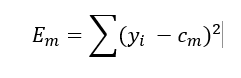Thus, the total error E is the sum of the errors in all segments m.
因此，总误差E是所有段m中的误差之和。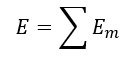We need to find S, so that the total error E is minimized.
我们需要找到S ，以使总误差E最小。
In this example, after running a brute force search for the optimal split S, that is calculating mean and error for the two segments with a moving point along the whole range of x, we found that the optimum split is S = 93.5. We used a brute force search strategy, but any other search strategy would have worked . This split S will become the root of the tree, as you can see in Figure 4.
在此示例中，在对最佳分割S进行蛮力搜索之后，即计算沿x整个范围移动的两个段的均值和误差，我们发现最佳分割为S = 93.5。 我们使用了蛮力搜索策略，但是任何其他搜索策略都可以使用。 如图4所示，此拆分S将成为树的根。
Then, we grow this tree by finding another split within each of the segments, in the same way as for the previous split. We continue this process in the branch until we reach one of these stopping criteria:
然后，通过与上一个拆分相同的方式，在每个段中找到另一个拆分来生长此树。 我们在分支中继续此过程，直到达到以下停止条件之一：
- if all points in a node have identical values for all input features
-如果节点中的所有点的所有输入要素都具有相同的值
- if the next split does not significantly reduce the total error
-如果下一次拆分并未显着减少总误差
- if a split produces a node smaller than the minimum node size
-如果拆分产生的节点小于最小节点大小
Limiting the minimum node size and the tree depth is important in order to avoid overfitting.
限制最小节点大小和树深度对于避免过度拟合很重要。
By following the splits on a regression tree, we can easily reach the predicted outcome. The resulting tree is shown in Figure 4.
通过遵循回归树上的拆分，我们可以轻松达到预期的结果。 生成的树如图4所示。
Figure 4. The final regression tree fitting MPG (y) from HP (x) on the Auto-MPG dataset.

评分指标 (Scoring Metrics)
Once you fit a regression model to your data, how can you evaluate how accurate your model is? There are several goodness-of-fit metrics for this, e.g.: the mean absolute error (MAE), the root mean squared error (RMSE), or the R-squared, just to name a few.
将回归模型拟合到数据后，如何评估模型的准确性？ 为此，有几个拟合优度度量，例如：平均绝对误差(MAE)，均方根误差(RMSE)或R平方，仅举几个例子。
The mean absolute error, or MAE, is calculated as the average of residuals. It is in the same scale as the target variable y, and it can be interpreted as the average deviation at each data point from the model.
平均绝对误差或MAE被计算为残差的平均值。 它与目标变量y具有相同的比例，并且可以解释为模型中每个数据点的平均偏差。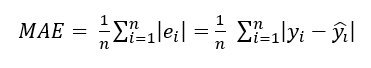The Root Mean Squared Error, or RMSE, is calculated as the name suggests — as the square root of the mean of squared residuals. Like MAE, it describes the deviation between the observed data and the model. However, due to its calculation, more weight is given to large deviations and consequently is more sensitive to such data points.
顾名思义，均方根误差(RMSE)的计算方式为残差均方根的平方根。 像MAE一样，它描述了观测数据与模型之间的偏差。 但是，由于其计算，较大的偏差将赋予更多的权重，因此对此类数据点更加敏感。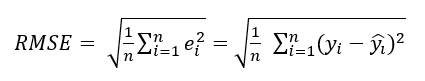R-squared is a relative measure of goodness-of-fit. It quantifies the proportion of the variability explained by the model. R-squared ranges from 0 to 1, with 0 indicating no variability explained by the model, and 1 indicating all variability explained by the model.
R平方是拟合优度的相对度量。 它量化了模型解释的可变性的比例。 R平方的范围是0到1，其中0表示模型没有解释变异性，而1表示模型解释了所有变异性。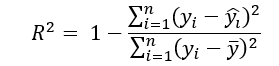In our toy problem, with the current set of parameters, we get the goodness-of-fit as in the following table.
在我们的玩具问题中，使用当前的参数集，我们得到了拟合优度，如下表所示。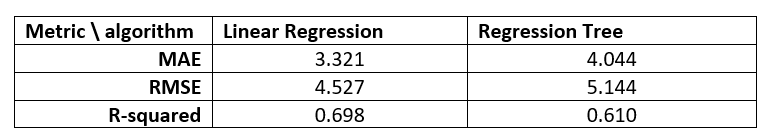利弊 (Pros and Cons)
Comparison on prediction error is not the only interesting comparison we can make. Indeed, one or the other algorithm will be best performing depending on the data and on the task.
比较预测误差并不是我们可以做的唯一有趣的比较。 实际上，根据数据和任务，一种或另一种算法将表现最佳。
In general, however, regression trees have a few advantages.
但是，总体而言，回归树具有一些优势。
- Ease of interpretation. We can go through the tree and clearly understand the decision process to assign one value or another to the input feature.
-易于解释。 我们可以遍历树，清楚地了解为输入要素分配一个值或另一个值的决策过程。
- Execution speed. Since most of the undesired data are filtered out at each step, the tree has to work on less data the further the creation of the tree proceeds. This also leads to independence from outliers.
-执行速度。 由于大多数不需要的数据在每个步骤中都会被过滤掉，因此树的创建越深入，树就必须处理更少的数据。 这也导致了离群值的独立性。
- No data preparation required. Because of the simple math used, it does not require statistical assumptions or special processing of the data. By comparison, linear regression requires normality of the outcome variable and independence of the training instances.
-无需数据准备。 由于使用了简单的数学运算，因此不需要统计假设或对数据进行特殊处理。 相比之下，线性回归需要结果变量的正态性和训练实例的独立性。
Linear regression has also some clear advantages.
线性回归也有一些明显的优势。
- Linearity. It makes the estimation procedure simple and easy to understand.
-线性。 它使估算程序简单易懂。
- On linearly separable problems of course it works best.
-当然，对于线性可分离的问题，效果最好。
So — as usual — depending on the problem and the data at hand, one algorithm will be preferable to the other.
因此，像往常一样，根据问题和手头的数据，一种算法将比另一种算法更可取。
The KNIME workflow used to train the linear regression model and the regression tree is shown in Figure 5 and available on the KNIME Hub under https://kni.me/w/gSAlDSojYMbi9wgl .
用于训练线性回归模型和回归树的KNIME工作流程如图5所示，可在KNIME Hub上的https://kni.me/w/gSAlDSojYMbi9wgl下找到。
We hope that this comparison has been useful to show and understand the main differences between linear regression and regression trees.
我们希望这种比较有助于显示和理解线性回归和回归树之间的主要区别。
Figure 5. The KNIME workflow used to generate the regression tree and the linear regression fitting MPG (y) from HP (x) on the Auto-MPG dataset, available on the KNIME Hub at
https://kni.me/w/gSAlDSojYMbi9wgl .

翻译自: https://medium.com/analytics-vidhya/basic-regression-models-5153454fe62f

建立统计回归模型的基本步骤

展开全文python 机器学习 人工智能 深度学习 大数据
• 建立线性回归模型的完整步骤 目录零、案例简介一、数据诊断1 正态性检验1.1 检验方法1.1.1 直方图法1.1.2 PP图与QQ图1.1.3 Shapiro检验和K-S检验1.2 校正方法2 多重共线性检验2.1 检验方法2.1.1 方差膨胀因子VIF2.2 ...目录

O、简述
一、建立模型
二、模型检验

1 模型的显著性检验——F检验
2 模型回归系数的显著性检验——t检验

三、数据诊断

1 正态性检验

1.1 检验方法

1.1.1 直方图法
1.1.2 PP图与QQ图
1.1.3 Shapiro检验和K-S检验

1.2 校正方法

2 多重共线性检验

2.1 检验方法

2.1.1 方差膨胀因


展开全文• 线性回归模型建模步骤 （一元线性回归、多元线性回归）
•matlab
• ## MATLAB建立回归模型

千次阅读 2020-01-11 11:13:40
终于考完数值分析和数理统计了，回来更新MATLAB 回归分析 1.一元线性回归 例如 有下列两组数据 x=1:10; y=[2650,1942,1493,1086,766,539,485,291,224,202];
终于考完数值分析和数理统计了，回来更新MATLAB 回归分析 在MATLAB的绘图里面其实有工具箱可以直接拟合数据，不过我们还是来看下代码简单拟合的方式。 1.一元线性回归 例如 有下列两组数据 x=1:10; y=[2650,1942,1493,1086,766,539,485,291,224,202]; 我们先用线性回归的思路，先画出数据的散点图
clear all
clc
%做x和y的散点图%
x=1:10;
y=[2650,1942,1493,1086,766,539,485,291,224,202];
for i=1:10
plot(x(i),y(i),'ok');
hold on
end
xlabel('x');
ylabel('y');

我们可以从图中看出不是成线性的，而是近似和对数函数相似，所以我们使用对数函数进行拟合
x=1:10;
y=[2650,1942,1493,1086,766,539,485,291,224,202];
z=zeros(size(y));
N=length(y);
for i=1:N
z(i)=log(y(i));
plot(x(i),z(i),'ok');
hold on
end
xlabel('x');
ylabel('y');


实际运行之后可以看粗已经近似与一条直线，故我们再编写代码直接求出回归系数：
x=1:10;
y=[2650,1942,1493,1086,766,539,485,291,224,202];
z=zeros(size(y));
N=length(y);
for i=1:N
z(i)=log(y(i));
end
[p,s]=polyfit(x,z,1)

一元线性回归到这里结束 我们接下来看下多元线性回归
[b,bint,r,rint,status]=regress(y,x,alpha)

例如有以下数据 x1=[1.376, 1.375, 1.387, 1.401, 1.412, 1.428, 1.445, 1.477]; x2=[0.450,0.475,0.485,0.500,0.535,0.545,0.550,0.575]; x3=[2.170,2.554,2.676,2.713,2.823,3.088,3.122,3.262]; x4=[0.8922, 1.1610,0.5346,0.9589, 1.0239, 1.0499,1.1065, 1.1387]; y=[5.19, 5.30,5.60,5.82,6.00,6.06,6.45,6.95];
这可以直接理解为一个矩阵方程x为系数，y为函数值，与正交二项式的线性拟合方式类似，MATLAB求解即可
clear all
clc
x1=[1.376, 1.375, 1.387, 1.401, 1.412, 1.428, 1.445, 1.477];
x2=[0.450,0.475,0.485,0.500,0.535,0.545,0.550,0.575];
x3=[2.170,2.554,2.676,2.713,2.823,3.088,3.122,3.262];
x4=[0.8922, 1.1610,0.5346,0.9589, 1.0239, 1.0499,1.1065, 1.1387];
y=[5.19, 5.30,5.60,5.82,6.00,6.06,6.45,6.95];
save data x1 x2 x3 x4 y
Y=[y'];
x=[ones(size(x1')),x1',x2',x3',x4'];
% x=[ones(size(x1')),x1',x2',x3',x4'];
[b,bint,r,rint,stats]=regress(Y,x)

写到这里，谢谢观看`
展开全文• 向量自回归模型建模步骤梳理（VAR、VMA、VARMA模型）
• 本文简单介绍下，使用SPSSAU进行面板数据回归分析步骤。 一、数据格式 下图中，展示的就是一个面板数据的例子。数据为9个地区2008~2018共11年的各项经济指标数据。 地区列反映的是数据不同的截面，即不同的...
• 一、明确需求（因变量y） 二、数据清洗 缺失值处理 异常值处理：一般大于3倍标准差的值视为异常值 分类变量的处理：将分类变量改为多列进行处理 备注：异常值一般不要直接...五、针对训练集跑回归模型并检验 F...
• 目录 一、Excel 数据分析选项 二、线性回归分析 三、总结 四、参考资料 本文内容：对年龄-心率原始数据，用 Excel 做线性回归。 工具：Excel 2016——提取码：rirp 一、Excel 数据分析选项 下载安装包并安装，然后...excel
• 多重共线性检验通过样本数据建立回归方程一般不能直接立即用于对实际问题的分析和预测，需要进行各种统计检验，主要包括回归方程的拟合优度检验、回归方程显著性检验、回归系数显著性检验、残差分析、异常值检验和...
• 废话不多说，先开始分享： 1. 首先啥是VAR模型，我这里简略通俗的说一下，...但是VAR模型除了分析自身滞后项的影响外，还分析其他相关因素的滞后项对未来值产生的影响，模型的形式为： 其中就是其他因子的滞后项...python VAR
• 空间计量模型的matlab代码 包括OLS SLM SEM SDM SDEM
• 一个回归分析步骤： 1. 用scat x y 查看散点图 2. 使用适当的模型进行回归分析 ls 3. 时间序列数据要做自相关性分析，横截面数据做异方差性检验先找到数据，巧妇难为无米之炊； 如果找不到数据，试着把问题...计量经济学 自相关性
• 清楚了回归分析的目的后，下面我们以回归分析预测法的步骤来说明什么是回归分析法： 　回归分析是对具有因果关系的影响因素（自变量）和预测对象（因变量）所进行的数理统计分析处理。只有当变量与因变量确实存在......